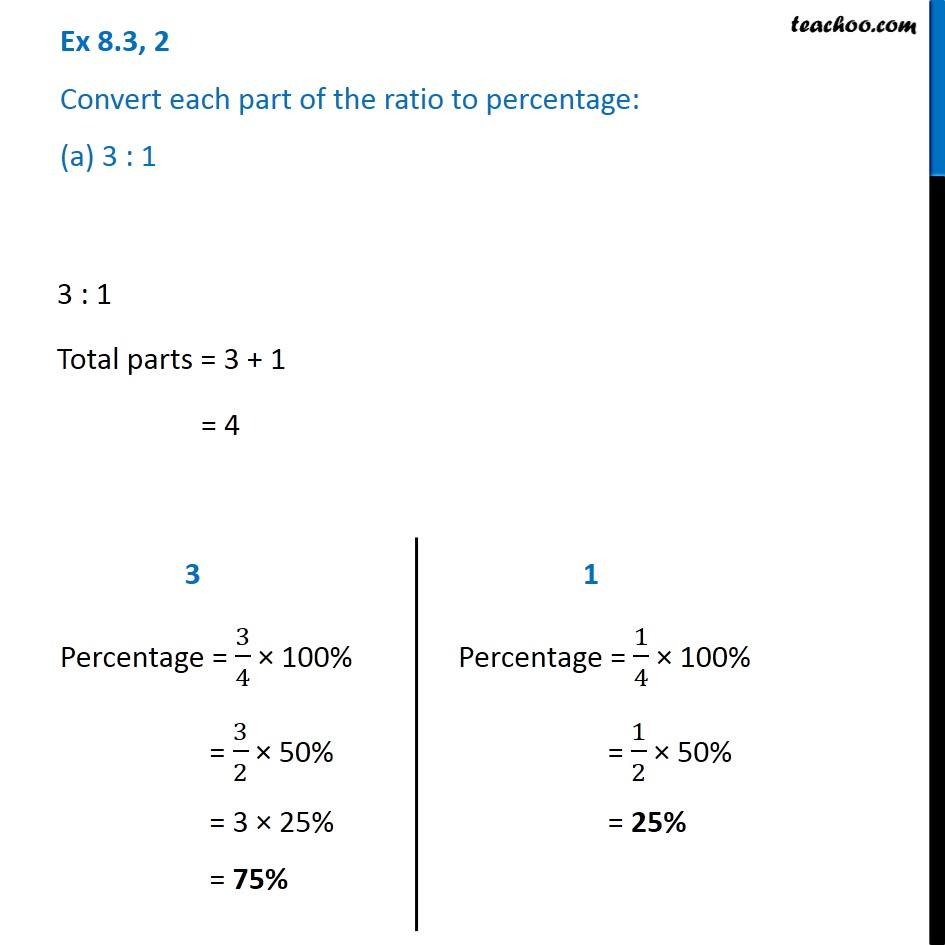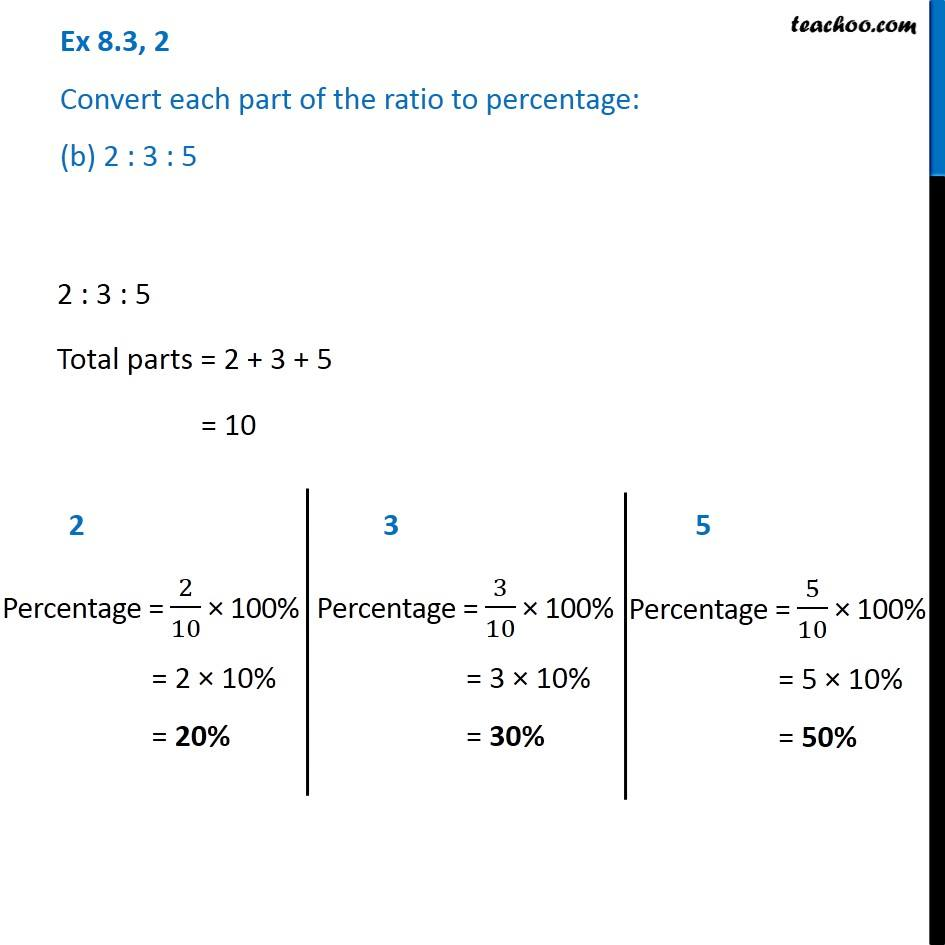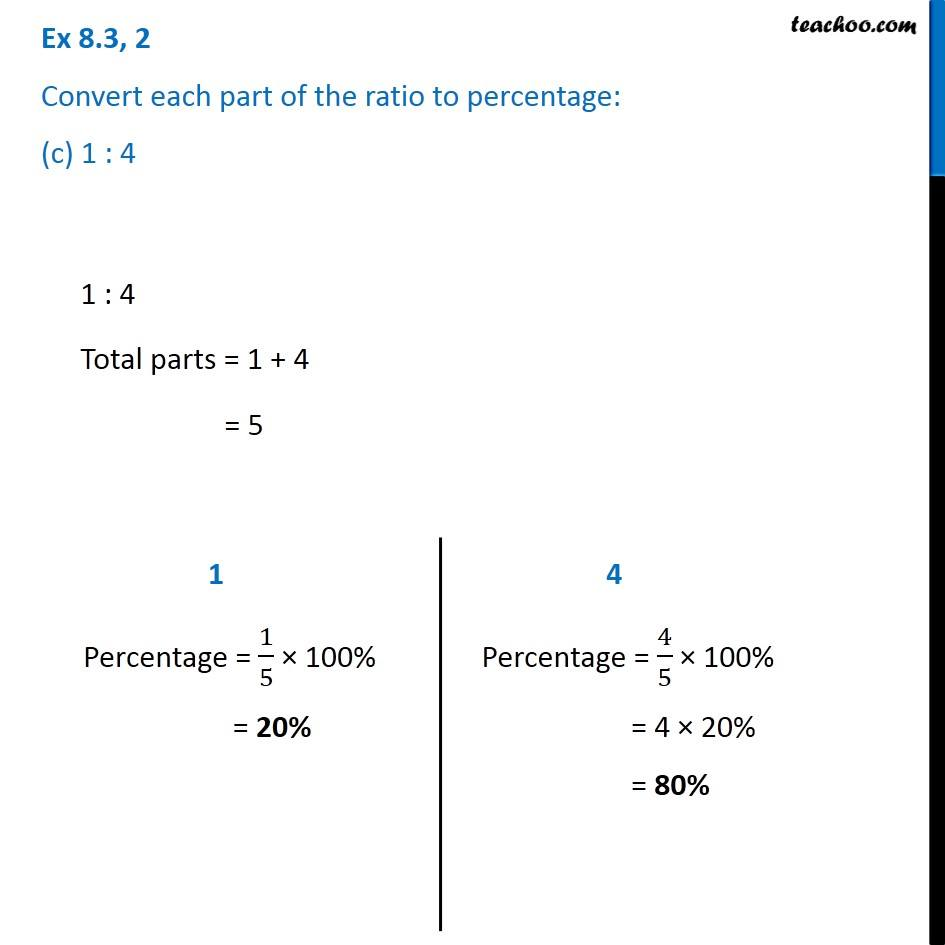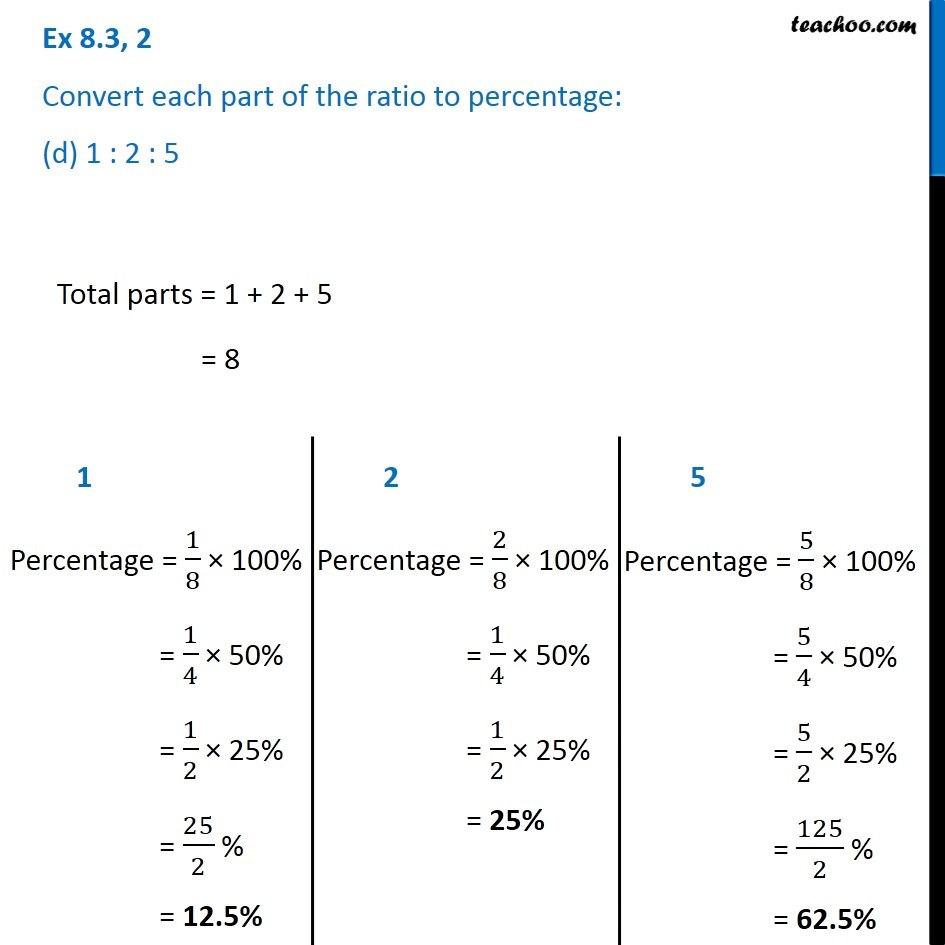Subscribe to our Youtube Channel - https://you.tube/teachoo

1. Chapter 8 Class 7 Comparing Quantities
2. Serial order wise
3. Ex 8.3

Transcript

Ex 8.3, 2 Convert each part of the ratio to percentage: (a) 3 : 1 3 : 1 Total parts = 3 + 1 = 4 3 Percentage = 3/4 × 100% = 3/2 × 50% = 3 × 25% = 75% 1 Percentage = 1/4 × 100% = 1/2 × 50% = 25% Ex 8.3, 2 Convert each part of the ratio to percentage: (b) 2 : 3 : 5 2 : 3 : 5 Total parts = 2 + 3 + 5 = 10 2 Percentage = 2/10 × 100% = 2 × 10% = 20% 3 Percentage = 3/10 × 100% = 3 × 10% = 30% 5 Percentage = 5/10 × 100% = 5 × 10% = 50% Ex 8.3, 2 Convert each part of the ratio to percentage: (c) 1 : 4 1 : 4 Total parts = 1 + 4 = 5 1 Percentage = 1/5 × 100% = 20% 4 Percentage = 4/5 × 100% = 4 × 20% = 80% Ex 8.3, 2 Convert each part of the ratio to percentage: (d) 1 : 2 : 5 Total parts = 1 + 2 + 5 = 8 1 Percentage = 1/8 × 100% = 1/4 × 50% = 1/2 × 25% = 25/2 % = 12.5% 2 Percentage = 2/8 × 100% = 1/4 × 50% = 1/2 × 25% = 25% 5 Percentage = 5/8 × 100% = 5/4 × 50% = 5/2 × 25% = 125/2 % = 62.5%

Ex 8.3